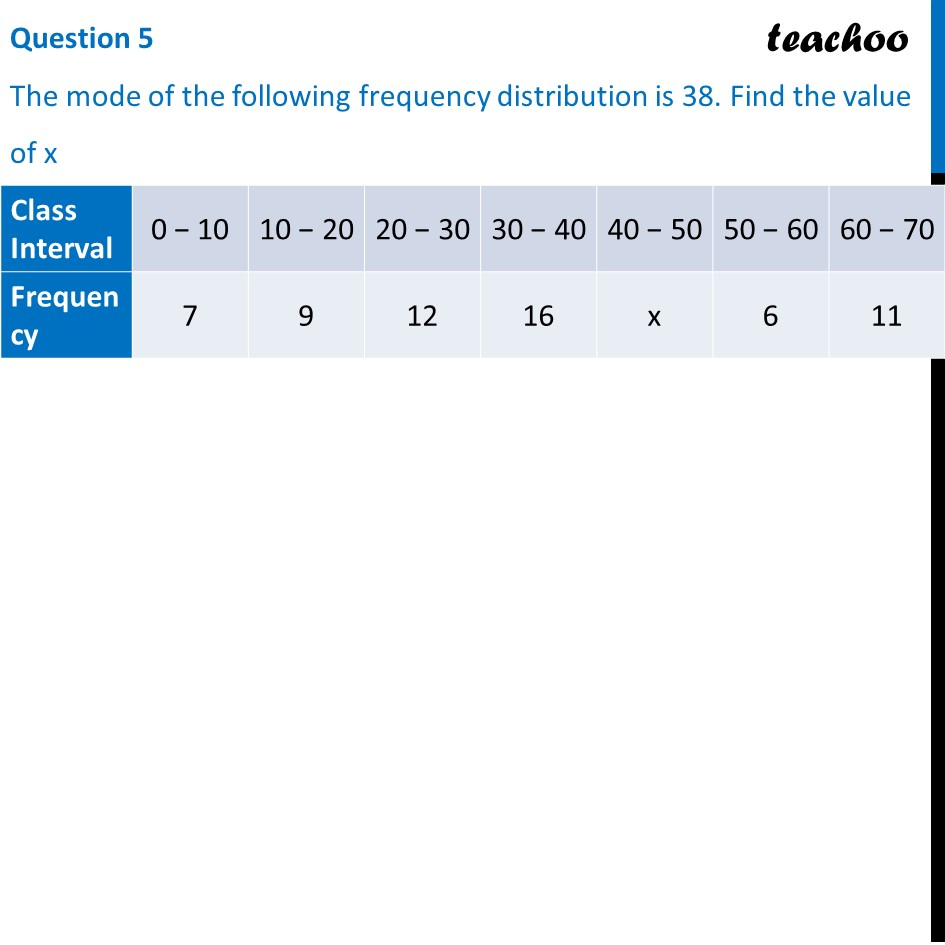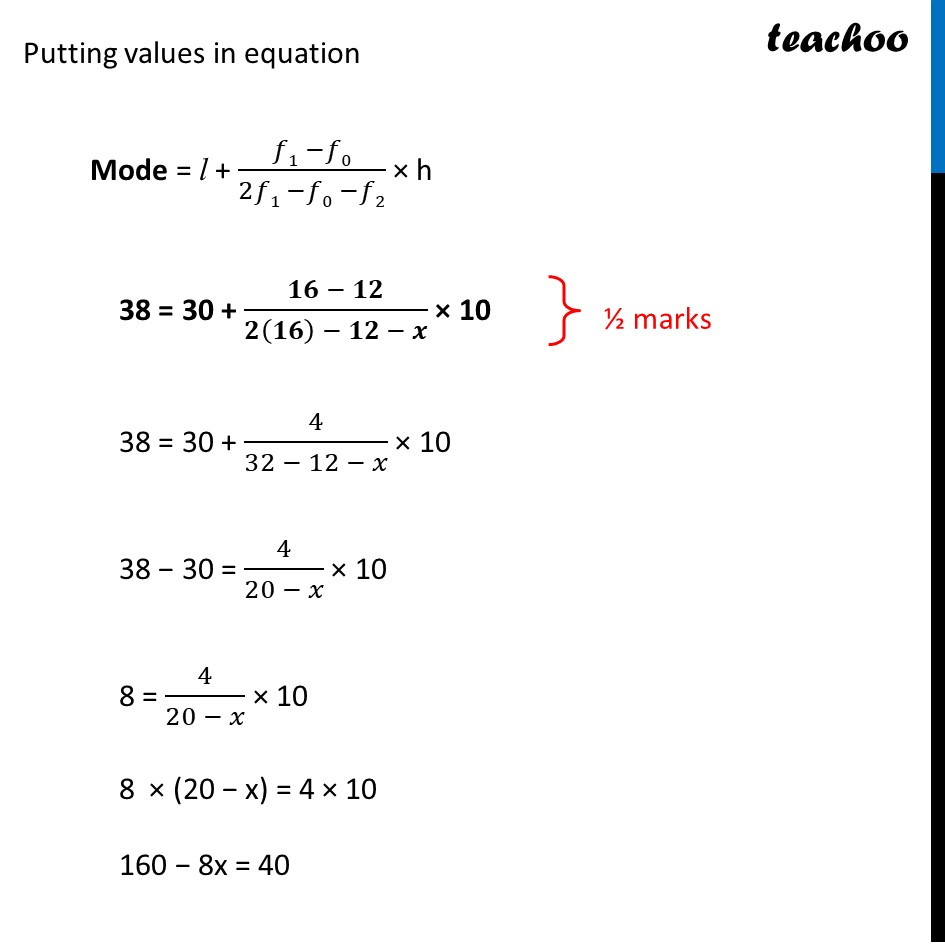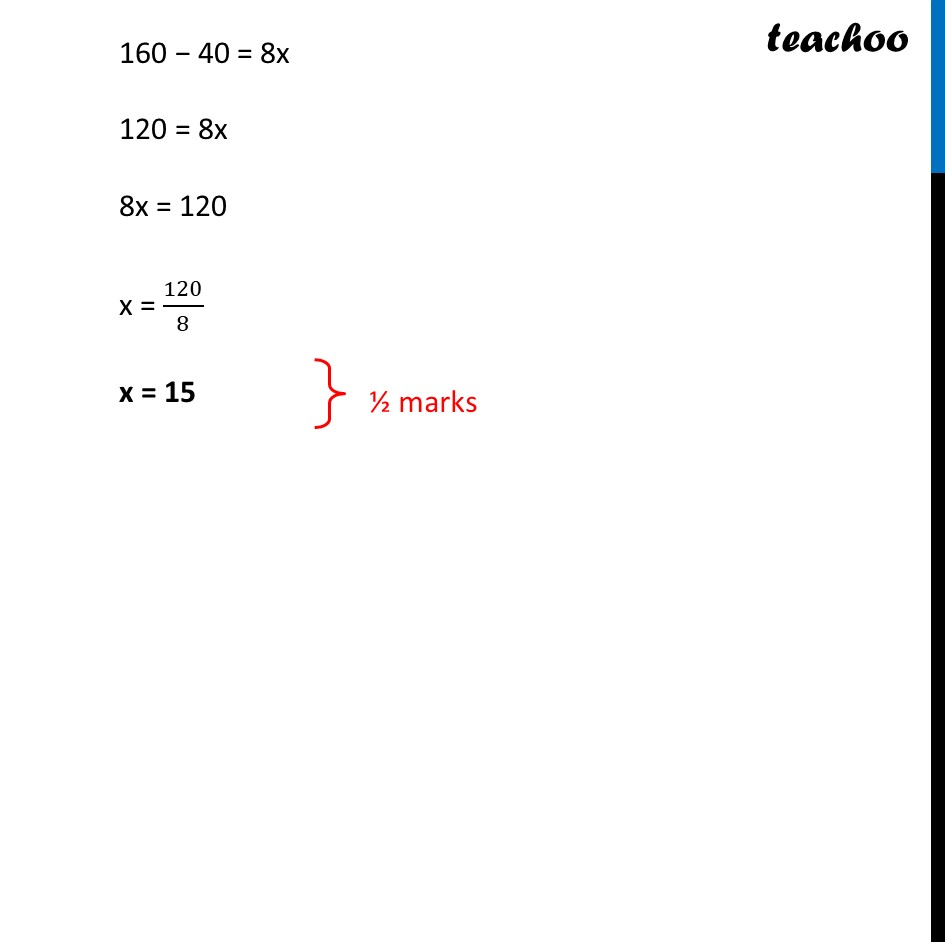CBSE Class 10 Sample Paper for 2022 Boards - Maths Basic [Term 2]

Class 10
Solutions of Sample Papers for Class 10 Boards

## An inter house cricket match was organized by a school. Distribution of runs made by the students is given below. Find the median runs scored.

This question is similar to Example 8 Chapter 14 Class 10 - StatisticsGet live Maths 1-on-1 Classs - Class 6 to 12

### Transcript

An inter house cricket match was organized by a school. Distribution of runs made by the students is given below. Find the median runs scored. Since Mode = 38 30 – 40 is Modal Class Now, Mode = l + (𝒇𝟏 −𝒇𝟎)/(𝟐𝒇𝟏 −𝒇𝟎 −𝒇𝟐) × h where l = lower limit of modal class h = class-interval f1 = frequency of the modal class f0 = frequency of class before modal class f2 = frequency of class after modal class Putting values in equation Mode = l + (𝑓1 −𝑓0)/(2𝑓1 −𝑓0 −𝑓2) × h 38 = 30 + (𝟏𝟔 − 𝟏𝟐)/(𝟐(𝟏𝟔) − 𝟏𝟐 − 𝒙) × 10 38 = 30 + 4/(32 − 12 − 𝑥) × 10 38 − 30 = 4/(20 − 𝑥) × 10 8 = 4/(20 − 𝑥) × 10 8 × (20 − x) = 4 × 10 160 − 8x = 40 160 − 40 = 8x 120 = 8x 8x = 120 x = 120/8 x = 15